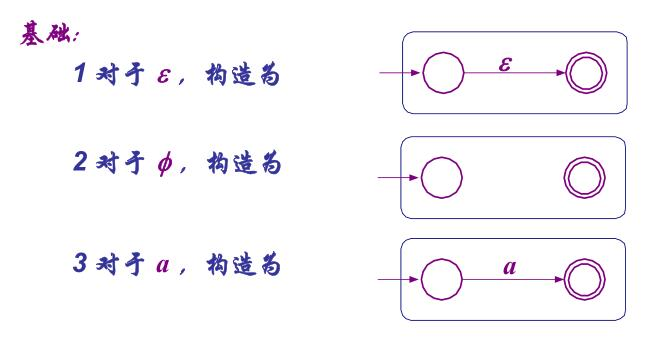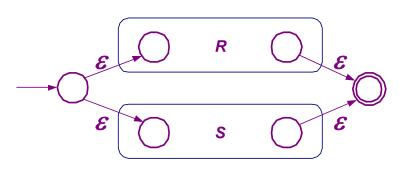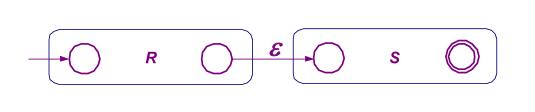# 右线性语言

## 正则集和正则式

• 正则集可用正则式表示。字母表 T 上的一个正则式和它表示的正则集可递归定义如下：
• ε 和 ∅ 都是正则式，分别表示的正则集是 {ε} 和空集 ∅；
• 任意 a ∈ T 是正则式，他表示的正则集是 {a}（以上两条为原子正则式）；
• 如果 A 和 B 是正则式，分别表示的正则集是 L(A) 和 L(B)，则 (A+B)、(A·B)、(A) 也都是正则式，分别表示的正则集是 `L(A) ∪ L(B)``L(A)L(B)`、`L(A)`。操作的优先级为`*（闭包） > ·（连接）> +（联合）`。
• 仅由 有限次 使用以上三步定义的表达式才是字母表 T 的正则式。如果两个正则式表示相同的正则集，则称两个正则式相等。
• 假设 α、β、γ 都是正则式，则：
• `(α + β) + γ = α + (β + γ)`
• `(α · β) · γ = α · (β · γ)`
• `α + β = β + α`
• `α + α = α`
• `α · (β + γ) = (α · β) + (α · γ)`
• `(β + γ) · α = (β · α) + (γ · α)`
• `α + ∅= α`（零元）
• `α · ∅ = ∅ · α = ∅`（零元）
• `α · ε = ε · a = a`（幺元）
• `(α*)* = α*`（闭包）
• `α* = ε + α+`
• `∅* = ε`
• 注意：
• 正则集是 T* 的子集；
• L+ 包含 ε 当且仅当 L 包含 ε；
• 每个正则集至少对应一个正则式。

## 右线性文法与正则

### 右线性文法与正则式

• 右线性文法（正则文法）与正则式具有等价性。
• 求解规则：设 `x = αx + β, α ∈ T*, β ∈ (N∪T)*, x ∈ N`，可解出 `x = α*β`
• 例：`G = {N = {S, A, B}, T = {a, b}, P, S}`，其中 P 包括以下规则：`S → aA, S → bB, S → b, A → bA, A → ε, B → bS`
• 将 P 中的推导式联立，可得到 `S = aA + bB + b``A =bA + ε``B = bS`
• 根据 `A = bA + ε`，应用求解规则得到 `A = b*`
• `A = b*``B = bS` 代入，得到 `S = ab* + bbS + b`，转换形式得到 `S = (bb)S + (ab* + b)`
• 在此应用求解规则得到 `S = (bb)*(ab*+b)`

### 右线性文法与正则集

• 正则集是由右线性文法产生的语言，二者是等同的 ，按照上面定义的正则集都是右线性文法产生的语言。
• 设字母表为 T。∅、{ε} 和 {a}（任意 a ∈ T）都是正则集，则 ∅、{ε} 和 {a} 都是右线性语言。它们分别对应的右线性文法是 `G∅ = ({S}, T, ∅, S)``G{ε} = ({S}, T, {S → ε}, S)` 以及 `G{a} = ({S}, {a}, {S → a}, S)`
• 可证明，设字母表 T 上有右线性语言 L1 和 L2，则 L1∪L2、L1L2 和 L1* 都是右线性语言。
• 证 L1 ∪ L2 为右线性语言：有右线性文法 `G = (N, T, P, S)`，其中 `N = N1 ∪ N2 ∪ {S}``P = P1 ∪ P2 ∪ {S → S1, S → S2}`，S ∉ N1 ∪ N2，是一个新的非终结符；
• 证 L1L2 为右线性语言：有右线性文法 `G = (N, T, P, S)`，其中 N = N1 ∪ N2，S = S1，生成式 P 为：若 A → αB ∈ P1 则 A → αB ∈ P，若 A → α ∈ P1，则 A → αS2 ∈ P，P2 ⊆ P。
• 证 L1* 是右线性语言：有右线性文法 `G = (N, T, P, S)`，其中 N = N1 ∪ {S}，S ∉ N1，S 是一个新非终结符，生成式 P 定义为：若 A → αB ∈ P1 则 A → αB ∈ P，若 A → α ∈ P1 则 A → αS ∈ P 且 A → α ∈ P，且 P 中包括 {S → S1, S → ε}。

## 正则表达式和有限自动机

• 有限自动机、右（左）线性文法和正则表达式都定义了同一种语言（正则语言）。
• 由 DFA 构造等价的正则表达式（状态消去法）:• 从 DFA 构造等价正则表达式的具体步骤如下：
• 对每一终态 q，依次消去除 q 和初态 q0 之外的其它状态；
• 若 q ≠ q0，最终可得到一般形式：`(R+ SU*T)*SU*`，如下图中左侧的自动机；
• 若 q = q0，最终可得到如图中右侧的自动机，对应正则式为 `R*`
• 最终的正则表达式为 每一终态对应的正则表达式之和（联合）• 从正则表达式构建等价的 ε-NFA，可根据如下几种基本组合子组合：• 对于 `R+S``RS` 以及 `R*`，分别为下图中从上到下三种连接方式：## 右线性文法与有限自动机

### 右线性文法 ⇒ 有限自动机

• 设右线性文法 G = (N, T, P, S)，产生语言为 L(G)，则存在一个有限自动机 M 接受语言 L(M) = L(G)。
• 构造：构造一个与 G 等价的 NFA M = (Q, T, δ, q0, F)，其中
• Q = N ∪ {H}，H 为新增状态，H ∉ N；
• q0 = S；
• 当 S → ε ∈ P 时，F = {H, S}，否则 F = {H}；
• δ 的定义为：若 A → αB ∈ P 则 B ∈ δ(A, α)，若 A → α ∈ P 则 H ∈ δ(A, α)，且对任意输入有 δ(H, α) = ∅。
• 例：G = ({S, B}, {α, β}, P, S)，其中 P 为 S → αB, B → αB | βS | α。
• 令 NFA M = (Q, T, δ, q0, F)，Q = {S, B, H}，T = {α, β}，q0 = S，F = {H}；
• ∵ S → αB，故 δ(S, α) 中包括 B；
• ∵ B → αB，故 δ(B, α) 中包括 B；
• ∵ B → βS，故 δ(B, β) 中包括 S；
• ∵ B → α，故 δ(B, α) 中包括 H。

### 有限自动机 ⇒ 右线性文法

• 设 DFA M 接受的语言为 L(M)，则存在右线性文法 G，它产生的语言 L(G) = L(M)。
• 构造：设 M = (Q, T, δ, q0, F)，则令 G = (N, T, P, S)，其中
• N = Q，S = q0；
• 若 δ(A, α) = B 且 B ∉ F 则 A → αB ∈ P；
• 若 δ(A, α) = B 且 B ∈ F 则 A → α ∈ P，A → αB ∈ P。
• 对于 NFA，可以转换为等价的 DFA 再做相应构造。

TODO